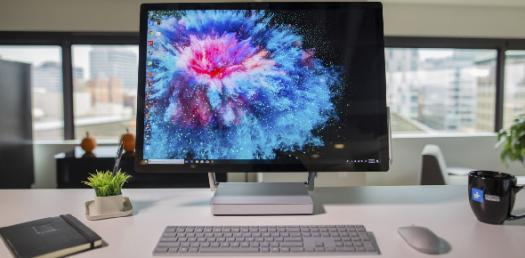The Ultimate Computer Quiz! From Easy To Hard Questions

20 Questions | Total Attempts: 185Settings.

• 1.
How many 3-line-to-8-line decoders are required for a 1-of-32 decoder?
• A.

1

• B.

2

• C.

4

• D.

8

• 2.
Race around condition may occur when J and K are
• A.

0,1

• B.

1,0

• C.

0,0

• D.

1,1

• 3.
One example of the use of an S-R flip-flop is as a(n):
• A.

Binary storage register

• B.

Racer

• C.

Astable oscillator

• D.

Transition pulse generator

• 4.
The primary use for Gray code is:
• A.

Coded representation of a shaft's mechanical position

• B.

Turning on/off software switches

• C.

To represent the correct ASCII code to indicate the angular position of a shaft on rotating machinery

• D.

To convert the angular position of a shaft on rotating machinery into hexadecimal code

• 5.
Why is the fan-out of CMOS gates frequency dependent?
• A.

Each CMOS input gate has a specific propagation time and this limits the number of different gates that can be connected to the output of a CMOS gate.

• B.

When the frequency reaches the critical value, the gate will only be capable of delivering 70% of the normal output voltage and consequently the output power will be one-half of normal; this defines the upper operating frequency.

• C.

The higher the number of gates attached to the output, the more frequently they will have to be serviced, thus reducing the frequency at which each will be serviced with an input signal.

• D.

The input gates of the FETs are predominantly capacitive, and as the signal frequency increases the capacitive loading also increases, thereby limiting the number of loads that may be attached to the output of the driving gate.

• 6.
• A.

Determine sign and magnitude

• B.

Reduce propagation delay

• C.

Add a 1 to complemented inputs

• D.

Increase ripple delay

• 7.
What is a shift register that will accept a parallel input, or a bidirectional serial load and internal shift features, called?
• A.

Tristate

• B.

End round

• C.

Universal

• D.

Conversion

• 8.
How can parallel data be taken out of a shift register simultaneously?
• A.

Use the Q output of the first FF.

• B.

Use the Q output of the last FF.

• C.

Tie all of the Q outputs together.

• D.

Use the Q output of each FF.

• 9.
When using even parity, where is the parity bit placed?
• A.

Before the MSB

• B.

After the LSB

• C.

In the parity word

• D.

After the odd parity bit

• 10.
A settable flip-flop's normal starting state when power is first applied to a circuit is always the ________ state.
• A.

Reset

• B.

Set

• C.

Toggle

• D.

Dual

• 11.
Why is a pull-up resistor needed for an open collector gate?
• A.

To provide Vcc for the IC

• B.

To provide ground for the IC

• C.

To provide the HIGH voltage

• D.

To provide the LOW voltage

• 12.
Which logic family is characterized by a multiemitter transistor on the input?
• A.

TTL

• B.

CMOS

• C.

ECL

• D.

None of the above

• 13.
What is one disadvantage of an S-R flip-flop?
• A.

It has no enable input

• B.

It has an invalid state

• C.

It has no clock input

• D.

It has only a single output

• 14.
How many flip-flops are required to construct a decade counter?
• A.

10

• B.

8

• C.

5

• D.

4

• 15.
MOD-6 and MOD-12 counters are most commonly used as:
• A.

Frequency counters

• B.

Multiplexed displays

• C.

Digital clocks

• D.

Power consumption meters

• 16.
Which of the following logic families has the highest maximum clock frequency?
• A.

S-TTL

• B.

AS-TTL

• C.

HS-TTL

• D.

HCMOS

• 17.
Which of the following logic families has the shortest propagation delay?
• A.

S-TTL

• B.

AS-TTL

• C.

HS-TTL

• D.

HCMOS

• 18.
Which of the logic families listed below allows the highest operating frequency?
• A.

74AS

• B.

54S

• C.

ECL

• D.

HCMOS

• 19.
Which search engine was named KUMO during its internal testing?
• A.

• B.

Yahoo

• C.

Bing

• D.

Baidu

• 20.
Who is the author of "Fault Lines : How hidden fractures still threaten the world economy" ?
• A.

Manmohan Singh

• B.

D.Subba Rao

• C.

Raghuram Rajan

• D.

Amitav Ghosh

• 21.
In the digital clock project, what type of counter is used to count to 59 seconds?
• A.

MOD-60

• B.

MOD-6

• C.

BCD

• D.

BCD followed by MOD-6

• 22.
Who is the only Indian to have been awarded the title 'Hero of the Soviet Union' ?
• A.

Rakesh Sharma

• B.

Abdul Kalam

• C.

Ravish Malhotra

• D.

• 23.
GT, Antara,Insignia and Ampera are key brands of which company?
• A.

Renault

• B.

Opel

• C.

Daewoo

• D.

BMW

• 24.
Who is the first Indian to win a medal in Olympics in Badminton?
• A.

Pullela Gopichand

• B.

Saina Nehwal

• C.

Parupalli Kashyap

• D.

P.V. Sindhu

• 25.
Arachnophobia refers to the fear of ______________?
• A.

Cockroaches

• B.

Spider

• C.

Honey bees

• D.

Ants

Related TopicsBack to top